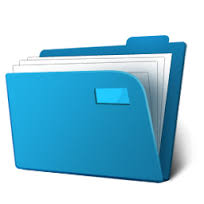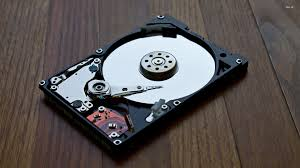# Basics Of File Size Conversion & KB v/s MB v/s GB Explained In DepthLet’s take real time example to explain about files. We all know how files look whether it is digital or a physical the common thing in these both types of file is that it contains data and have a size too. A physical file that we carry normally the ones with paper pages can be expressed in size with a number of pages. But the digital file that we store in our system represents term size in terms of bits and bytes.If you have confusion in the understanding difference between bits and bytes and that will be explained too.

### Understanding Basics Of File Size Conversion

So, File can be expressed as a collection of data which can be a collection of alphabets, numbers, symbols or some mathematical formulas and expression whose size is expressed in terms of bits , bytes, kilobits, kilobytes and so on. Which is the amount of space it consumes in filesystem.

### Understanding Bits & Bytes:

Defining Byte then we can say that 1 Byte contains 8 bits which mean a Byte contain a sequence of 8 bits. 1 Byte is enough to represent one alphanumeric character. A single character consumes at least 1 Byte which equals 8 bits.

1 Byte = 8 bits

If you are still confused in understanding bits or Bytes then try this. Bytes is always represented with uppercase “B” and bites is represented with lowercase “b”. So when you are reading these terms just recognize uppercase and lowercase of starting letters so you can become more confident about understanding file sizes.

###Nowadays hard drives are measured in GB(Gigabyte) and TB(Terabyte). So it is easy to know how much amount of space your hard disk carries actually. We prefer at least 1 TB of the hard drive for storing all our stuff like music, movies, files etc. But if you have 1 TB of hard disk then you will not get complete 1 TB of space on your disk you will get 995 GB of space because some portion is reserved for hard drive driver. And same goes for some peripheral devices like external hard disk and pen drives so you don’t get the exactly specified amount of space but some less.

### Understanding KB, MB & GB:

Now comes the main understanding part. Most of us get confused in kilobit & kilobyte , megabit & megabyte also gigabit & gigabyte. There are so many terms that make you go crazy so , I will explain you this in a very detailed manner and once you understand you can easily sort the differences between KB, MB & GB also much more.

1 Kilobit(Kb) = 1,024 bits

1 Megabit(Mb) = 1,024 kilobits (Kb)

1 Gigabit(Gb) = 1,024 megabits (Mb)

Note: Observe the difference between kilobyte which is represented as “KB” and Kilobit which is represented as “Kb”.

Similarly let us see for kilobytes(KB) , megabytes(MB) and gigabytes(GB)

1 KB = 1,024 Bytes

1 MB  = 1,000 KB

1 GB = 1,000 MB

Even terms like Terabytes(TB) and Petabytes(PB) are very common in terms of space now. So, we can also understand differences between them.

1 TB = 1,000 GB

1 PB = 1,000 TB

### KB v/s MB v/s GB:

Since we have already discussed the basics of kb,mb & gb to get you understand in a brief let us have a look at the table for more description.### Measure Of Data Transfer Speed:

Data transfer speed over a network which can be a LAN or WiFi is calculated in terms of bits per second. Higher the value of kb more data can be transferred. Which ultimately increases the speed of a network.

1 kbps (kilobits per seconds) = 1000 bits per second

1 Mbps (megabits per second) = 1000 kilobits per second

1 Gbps (gigabits per second) =  1000 megabits per second